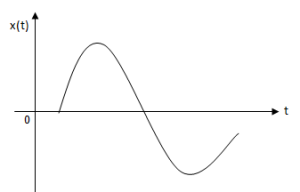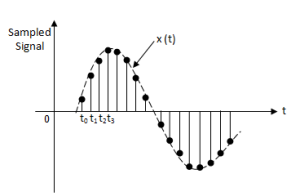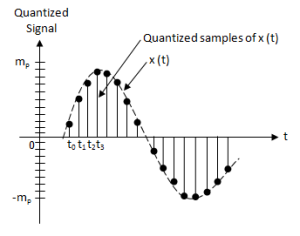# Concept of Quantization

In communication systems, sometimes it happens that we are available with analog signal, however, we have to transmit a digital signal for a particular application . In such cases, we have to convert an analog signal into digital signal .

This means that we have to convert a continuous time signal in the form of digits .

To see how a signal can be converted from analog to digital form, let us consider an analog signal as shown in fig.1 .Fig. 1 : Analog signal

First of all, we get samples of this signal according to sampling theorem .

For this purpose, we mark the time-instants t0 , t, t2  and so on , at equal time-intervals along the time axis .

At each of these time-instants the magnitude of the signal is measured and thus samples of the signal are taken .

Fig.2 shows a representation of the signal of fig.1 in terms of its samples .Fig . 2 : Samples of analog signal

Now, we can say that the signal in fig.2 is defined only at the sampling instants . This means that it no longer is a continuous function of time, but rather , it is a discrete-time signal .

However, since the magnitude of each sample can take any value in a continuous range, the signal in fig.2 is still an analog signal .

This difficulty is neatly resolved by a process known as quantization .

In quantization, the total amplitude range which the signal may occupy is divided into a number of standard levels .Fig.3 : Quantization

As shown in fig.3 , amplitudes of the signal x(t) lie in the range ( – m,  m) which is partitioned into L intervals, each of magnitude :

Δv = 2mp/ L

Now, each sample is approximated or rounded off to the nearest quantization level as shown in figure . Since each sample is now approximated to one of the L numbers therefore the information is digitized .